## Main

The difficulty of finding the ground-state properties of a large system of interacting electrons originates both from the exponential dimension of the underlying Hilbert space and from the fermionic nature of the wavefunction. It is a problem encountered virtually everywhere in quantum chemistry as well as in condensed-matter physics: for instance, the spatial configuration of a molecule is the one for which the energy of the interacting electrons moving in the nuclear potential, together with the electrostatic energy of the nuclei, becomes minimal. Similarly, a rich variety of phenomena in solid-state physics, in particular conductance and magnetic phenomena, can be understood by considering electrons moving in the periodic lattice potential, including such exciting phenomena as high-temperature superconductivity and the fractional quantum Hall effect.

A system of N electrons is described by the Hamiltonian(γ>0, and xi=(ri,si) with ri position and si spin), where the potential V contains both an electrostatic field φ(r) and a magnetic field B(r), which couples to the spin (the coupling to the orbit can be ignored for our purposes; see Supplementary Information), and the problem is to find the ground state within the set of fermionic (that is, antisymmetric) quantum states. Following the early work of ref. 3, it was shown1,2 that this problem could be rephrased as a single-particle minimization problem, for the reason that the only problem-dependent part is the external potential V, whose expectation value only depends on the local density, whereas the kinetic and interaction terms T and I are fixed and universal for all systems. Thus, the ground-state energy is given bywhere ρ is a single-electron density, and the functional F contains the problem-independent minimization over T and I,Here, the minimization runs over all N-electron density operators Ω that give rise to the reduced density ρ. The central requirement for a good density functional theory (DFT) algorithm is to find a suitable approximation to the universal functional, and indeed better and better techniques have been developed, making DFT the most widely used and most successful algorithm for treating interacting electrons.

However, as we show in this letter, there exist fundamental limits that constrain the ability to find a generally applicable and efficiently computable approximation to the universal functional, and thus put bounds on the applicability of DFT. To this end, we consider the two-dimensional (2D) Hubbard model with local magnetic fields, which arises from the problem of interacting electrons for a specifically chosen lattice potential, and can thus be simulated using DFT. We first determine the computational complexity of solving the Hubbard model and show that it is among the hardest problems in the complexity class QMA, Quantum Merlin Arthur. QMA contains problems that are believed to be hard to solve even by quantum computers, but once a solution is found it can be checked efficiently by a quantum computer. Thus, QMA encompasses the complexity class NP. We compare this with the difficulty of solving the Hubbard model using DFT with a suitable approximation of the functional at hand, and find that in that case the Hubbard model can be solved by a classical computer in a time polynomial in the number of electrons. This means that the existence of an efficient approximation to the functional would imply QMA=P, that is, computing the functional to polynomial accuracy in the number of electrons is a QMA-hard problem, which poses fundamental limitations on the ability to approximate the functional in DFT. Of course, this does not mean that DFT is not applicable in practice: much lower (for example constant) accuracies will typically suffice, and DFT is indeed a highly successful method.

The 2D Hubbard model4,5 describes a system of fermions hopping on a lattice. Although it typically appears as a phenomenological model for strongly bound electrons in solid-state physics6, it can be derived rigorously from (1) for an appropriate potential, as we show in the Supplementary Information. The Hubbard model with local magnetic fields is given by the Hamiltonian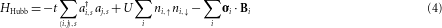where ai,s creates an electron of spin s{↑,↓} on lattice site i, 〈i,j〉 denotes nearest neighbours on the 2D square lattice, n=aa, σi=(σx,i,σy,i,σz,i) andwiththe Pauli matrices. The first term describes an electron tunnelling at rate t from one site to the adjacent one without changing its spin and the second the on-site Coulomb repulsion U between two electrons of different spin sitting on the same site, and the rightmost term contains the contribution from the magnetic field, which imposes a local field Bi at each site i—this is the only term that we can tune locally.

The 2D Hubbard model is of high interest on its own, as it is the minimal model that is believed to describe the physics arising in high-temperature superconductivity, quantum magnetism and heavy fermions. Indeed, it is one of the most intensively studied models in solid-state physics, making the investigation of its computational complexity interesting on its own. In the following, we show that computing its ground-state energy up to polynomial accuracy is complete for the complexity class QMA, the quantum analogue of NP. (See Fig. 1 for the relation of the relevant complexity classes.) A decision problem is in QMA if—although possibly hard to solve even by a quantum computer—every positive instance has a quantum proof that can be checked efficiently by a quantum computer. In particular, finding the ground-state energy of a local spin system with an accuracy polynomial in the lattice size is in QMA: the ground state serves as a proof, as expectation values of local Hamiltonians can be estimated efficiently. Conversely, it has been shown that any circuit verifying a QMA proof can be encoded as a ground-state problem7,8; that is, ground-state problems are QMA-complete. (A problem is called complete for a class if it is among the hardest problems in this class, that is, if any problem in the class can be reduced to it.) Using the same argument as before, finding the ground-state energy of the Hubbard model is inside QMA, because it can be mapped to a spin system through the Jordan–Wigner transform: this enables us to specify its ground state using spins, in such a way that it is possible to measure the ground-state energy efficiently9.

In the following, we show that the Hubbard model with magnetic fields is also a hard problem for QMA, and thus QMA-complete. To this end, we start from a class of Hamiltonians for which finding the ground-state energy is known to be QMA-complete—that is, as hard as finding the ground-state energy of any local Hamiltonian—and show that this problem can be reduced to finding the ground-state energy of the Hubbard model with local magnetic fields. This is accomplished by a sequence of reductions, each of which reduces the previous Hamiltonian problem to a more restricted class of Hamiltonians. Each step makes use of perturbation-theory constructions (so-called gadgets), such that the original Hamiltonian arises as the effective low-energy theory of the new Hamiltonian13,14.

We start off with the Hamiltonian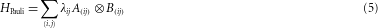defined on a 2D lattice with N spins, with A(i j) and B(i j) Pauli matrices and |λ|≤1, for which it has been proven that finding the ground-state energy up to a polynomial accuracy 1/q(N) is QMA-hard10. Following ref. 10, we call interactions of the form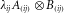Pauli interactions.

We first show how the Pauli Hamiltonian (5) can be reduced to the 2D Heisenberg lattice with local fields (see Supplementary Information for details). To this end, we use a chain of gadgets, all of which replace a two-qubit coupling by a chain of three qubits with a more restricted coupling. The idea is that by imposing a strong local field on the central (‘mediator’) qubit the system will essentially be in the ground state of the central qubit—but there will be second-order processes in which an excitation hops from the left qubit to the central one and then to the right, or vice versa, yielding an effective coupling between the outer qubits. (The excitation can also hop back and give an extra local term, which can however easily be compensated by adjusting the local magnetic field.) Note that similar gadgets have already been used, for example, in refs 10, 15.

The full sequence of reductions to the Heisenberg lattice is illustrated in Fig. 2. In a first step, we reduce arbitrary Pauli couplings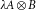to Pauli couplings with constant λ and AB. We illustrate this with a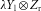coupling (we use X for σx and so on in the following), which is obtained from three qubits with couplings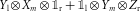by putting a strong field in the X Y plane on the central qubit: a short calculation shows that this indeed gives a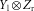coupling, where the strength is given by the angle in the X Y plane. The intuition behind this is that, for example, a Yl on the left qubit can excite the central qubit, and the excitation then hops to the right qubit as a Zr. In order for this hopping to be possible, the central field must not be along the X or Y axis, and correspondingly the hopping amplitude is controlled by the overlap of the field with X and Y eigenvectors.

The second gadget reduces Pauli couplings(AB) to Ising couplings. This is achieved by essentially the same gadget as before: for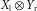, takeand place a strong field in X+Y direction on the m qubit. In a next gadget, Ising couplings are reduced to twocouplings: placing a strong Y field on the central qubits only allows for the hopping of excitations through the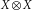part of the coupling. Similarly, the above coupling is reduced to the Heisenberg interaction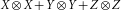: a strong Z field prohibits hopping through theterm, whereasdescribes hopping in the {|01〉,|10〉} subspace, which to second order yields the very same hopping term between the two outer qubits.

Putting these gadgets together, we have managed to reduce the QMA-complete Hamiltonian (5) to the Heisenberg Hamiltonian in a magnetic field on a sparse lattice. This can, in turn, be reduced to the full 2D Heisenberg lattice by using an ‘erasure gadget’, illustrated in Fig. 3: putting strong fields on the qubits to be erased decouples them up to polynomial precision. We have thus shown that the 2D Heisenberg Hamiltonian in a magnetic field on a 2D square lattice,is QMA-complete, both for J>0 (which we use further on) and J<0. Note that the presence of a magnetic field is crucial for the construction, as it is the only set of parameters available to encode a computational problem.Figure 3: The sparse Heisenberg lattice as obtained from H2D, equation (5), using a sequence of gadgets.

The final step is to reduce the Heisenberg lattice to the Hubbard model (4). The procedure can be found, for example, in ref. 6, and has been included in the Supplementary Information: in (4), we choose an on-site repulsion U very large as compared to t, and operate the system in the so-called half-occupancy regime where there are as many electrons as sites. (Note that this implies that a polynomial scaling in the lattice size is equal to a polynomial scaling in the number of electrons.) The tunnelling is suppressed as t/U, so that in the ground state each site will be occupied by exactly one electron, providing the desired spin degree of freedom. The coupling between the spins is achieved by a second-order process where one electron tunnels to an adjacent site, interacts with the other electron, and tunnels back. However, this can only take place if the spins form a singlet, giving rise to the effective Hamiltonian (6) up to a constant. As the process is of second order, we have that J=t2/U>0, and the error from higher-order processes is O(N3t3/U2); thus, U/t has to grow polynomially with the system size N.

All these gadgets can be combined straightforwardly: first, the gadgets in one layer do not interact, as they never share a coupling term. It can thus be checked straightforwardly that, for second-order perturbation theory, there will be no cross-talk between the gadgets. Second, all gadget layers can be applied one after another, as long as the total strength of the previous gadgets is sufficiently smaller than the strong local fields of the new gadgets. As the number of layers is constant, this can be achieved by choosing polyscale field strengths, which allows for a polynomial scaling of the interaction strength as well as an arbitrary polynomial precision in energy. (See Supplementary Information for details.)

Let us now turn our attention back to DFT and the problem of interacting electrons. As we show in the Supplementary Information, the Hubbard model (4) with arbitrary local fields arises from (1) for an appropriately chosen V. For this particular potential, we can explicitly write down the wavefunction wi(r) of each mode ai to sufficient precision. Thus, the ground-state wavefunction of the Hubbard model is supported by the wi(r), and consequently the single-electron density for the ground state of the Hubbard model must be of the form. As the functional (3) is convex and the physical λi,s,s form a convex set in, the minimization in equation (2) (up to polynomial accuracy) can be carried out efficiently16; that is, finding the ground-state energy of the Hubbard model is in P. This implies that PUF=QMA with UF an oracle for the universal functional, that is, computing the functional is QMA-hard under Turing reductions.

Let us note that there are alternative ways to define F[ρ], for example as the minimum over all pure N-electron states11,12, in which case F is not convex. Yet, efficient computability (or even certifiability) of F would still imply that we could give a certificate for the ground-state energy, that is, QMA would collapse to NP. This is considered very unlikely, thus implying that any reasonably defined F cannot be computed in NP.

Finally, DFT can also be based on a functional defined on two-electron densities, which can be computed efficiently11,12. In this case, the QMA-hardness of the problem arises from the fact that characterizing the set of allowed two-electron reduced states, the N-representability problem, is QMA-hard9; in fact, this provides an alternative proof of its hardness.